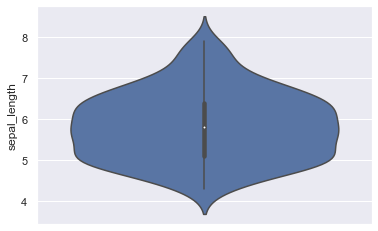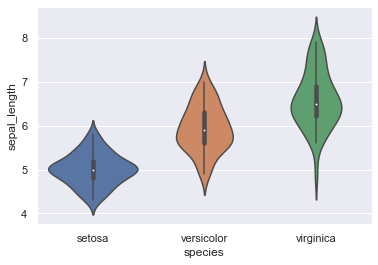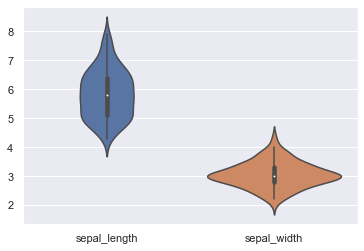## One numerical variable only

If you have only one numerical variable, you probably better have to make an histogram or a density plot. But you can still use the violinplot function to describe the distribution of this variable, as follows:

``````# libraries & dataset
import seaborn as sns
import matplotlib.pyplot as plt
# set a grey background (use sns.set_theme() if seaborn version 0.11.0 or above)
sns.set(style="darkgrid")

# Make boxplot for one group only
sns.violinplot(y=df["sepal_length"])
plt.show()``````## One variable and several groups

Usually, violinplots are used in cases similar to boxplots: when you have one numerical variable and several groups. It allows to compare distributions from one group to another. One usually works with two columns, one giving the value of the variable, the other one the group:

``````# libraries & dataset
import seaborn as sns
import matplotlib.pyplot as plt
# set a grey background (use sns.set_theme() if seaborn version 0.11.0 or above)
sns.set(style="darkgrid")

# plot
sns.violinplot( x=df["species"], y=df["sepal_length"] )
plt.show()``````## Several variables

Violinplots are also useful to compare several variables. In the iris dataset, we can compare the first 2 numerical variables:

``````# libraries & dataset
import seaborn as sns
import matplotlib.pyplot as plt
# set a grey background (use sns.set_theme() if seaborn version 0.11.0 or above)
sns.set(style="darkgrid")